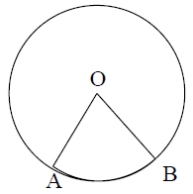# 1999COMC加拿大数学公开赛真题免费下载

### 真题与答案下载### 1999 COMC真题免费下载

Part A共8题，每题5分

Part B共4题，每题10分

#### 部分真题预览：

Part A :

6)trapezoid, DEFG, is circumscribed about a circle that has centre C and radius 2, as is shown. The shorter of the two parallel sides, DE, has length 3 and angles DEF and EFG are right angles. Determine the area of the trapezoid.7)The sector OAB of a circle, with centre O, has a perimeter of 12. Determine the radius of the circle which maximizes the area of the sector.8)Find the smallest positive integer k so that the expression (14k+17)/(k-9) becomes a fraction in the form pd/qd where p, q and d are positive integers, p and q have no common divisors, and neither q nor d equals 1.

Part B :

1)

1. Two identical triangles each have an area of 24. Their vertices are determined by the intersection of the lines with equations y = -4,x = 0 and y = (-3/4) x + b. Determine the two possible values for b.
2. For either of the two given triangles, a circle can be drawn to pass through its three vertices. What is the radius of this circle?

### 1999 COMC加拿大数学奥赛完整版答案免费下载